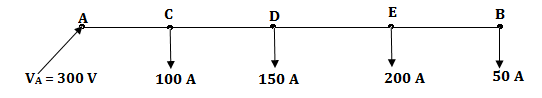# Power System - Transmission Distribution - Electrical Engineering (MCQ) questions and answers

1)   The ABCD constants of a 3 phase transposed transmission line with linear and passive elements ________________.

a. are always equal
b. never equal
c. only A and D are equal
d. only B and C are equal
 Answer  Explanation ANSWER: only A and D are equal Explanation: No explanation is available for this question!

2)   What is the empirical formula to calculate the number of strands?

a. 3n (n+2n) + 2
b. 3n (n+1) + 2
c. 3n (n+1) + 1
d. 3n (3+ 3n) + 1
 Answer  Explanation ANSWER: 3n (n+1) + 1 Explanation: No explanation is available for this question!

3)   What will be the pd at point B if a pd of 300 V is maintained at a point A, for a 2 wire dc distributor cable AB if 2 km long and supplies loads of 100A, 150A, 200A, and 50A suited at 500m, 1000m, 1600m, and 2000m from the feeding point A. Each conductor has a resistance of 0.01 Ω per 1000 m.a. 288 V
b. 287.6 V
c. 295.36 V
d. 291.2 V
 Answer  Explanation ANSWER: 287.6 V Explanation: No explanation is available for this question!

4)   Which type of insulators is used in guy wires?

a. Stay insulators
b. Shackle insulators
c. Pin type
d. Strain type
 Answer  Explanation ANSWER: Stay insulators Explanation: No explanation is available for this question!

5)   Where are the strain type insulators used?

b. At any intermediate anchor tower
c. On straight runs
d. Either (a) or (b)
 Answer  Explanation ANSWER: Either (a) or (b) Explanation: No explanation is available for this question!

6)   What is the safety factor of an insulator?

a. Puncture strength * Flash over voltage
b. Puncture strength / Flash over voltage
c. Flash over voltage / Puncture strength
d. None of these
 Answer  Explanation ANSWER: Puncture strength / Flash over voltage Explanation: No explanation is available for this question!

7)   Sag depends on which factor?

a. Span length
b. Weight of the conductors
c. Tension in the conductors
d. All of these
 Answer  Explanation ANSWER: All of these Explanation: No explanation is available for this question!

8)   In a balanced 3 phase, 4 wire ac system the phase sequence is RYB. If the voltage of R phase = 230 ∠ 0° volts, then what will be the B phase?

a. 230 ∠ - 120°
b. 230 ∠ - 90°
c. 230 ∠ - 90°
d. 230 ∠ - 120°
 Answer  Explanation ANSWER: 230 ∠ - 120° Explanation: No explanation is available for this question!

9)   The inductance of a power transmission line increases with

a. Decrease in line length
b. Increase in diameter of conductor
c. Increasing in spacing between the phase conductors
d. Increase in load current carried by the conductors
 Answer  Explanation ANSWER: Increasing in spacing between the phase conductors Explanation: No explanation is available for this question!

10)   What is the horizontal spacing between phase conductors of 132 kV line?

a. 8 m.
b. 11 m.
c. 14 m.
d. 17 m.
 Answer  Explanation ANSWER: 8 m. Explanation: No explanation is available for this question!

1 2 3 4 5 6 7 8 9 10 ...